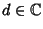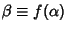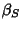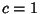## Möbius Transformation

A transformation of the formwhere,,,andis a Conformal Transformation and is called a Möbius transformation. It is linear in bothand.

Every Möbius transformation excepthas one or two Fixed Points. The Möbius transformation sends Circles and lines to Circles or lines. Möbius transformations preserve symmetry. The Cross-Ratio is invariant under a Möbius transformation. A Möbius transformation is a composition of translations, rotations, magnifications, and inversions.

To determine a particular Möbius transformation, specify the map of three points which preserve orientation. A particular Möbius transformation is then uniquely determined. To determine a general Möbius transformation, pick two symmetric pointsand. Define, restrictingas required. Compute.then equalssince the Möbius transformation preserves symmetry (the Symmetry Principle). Plug inandinto the general Möbius transformation and set equal toand. Without loss of generality, letand solve forandin terms of. Plug back into the general expression to obtain a Möbius transformation.